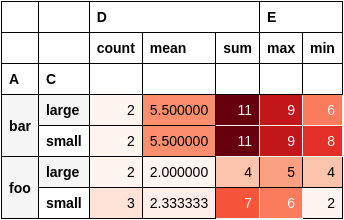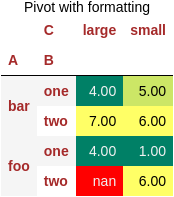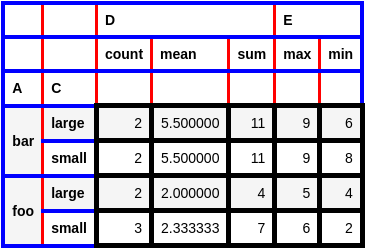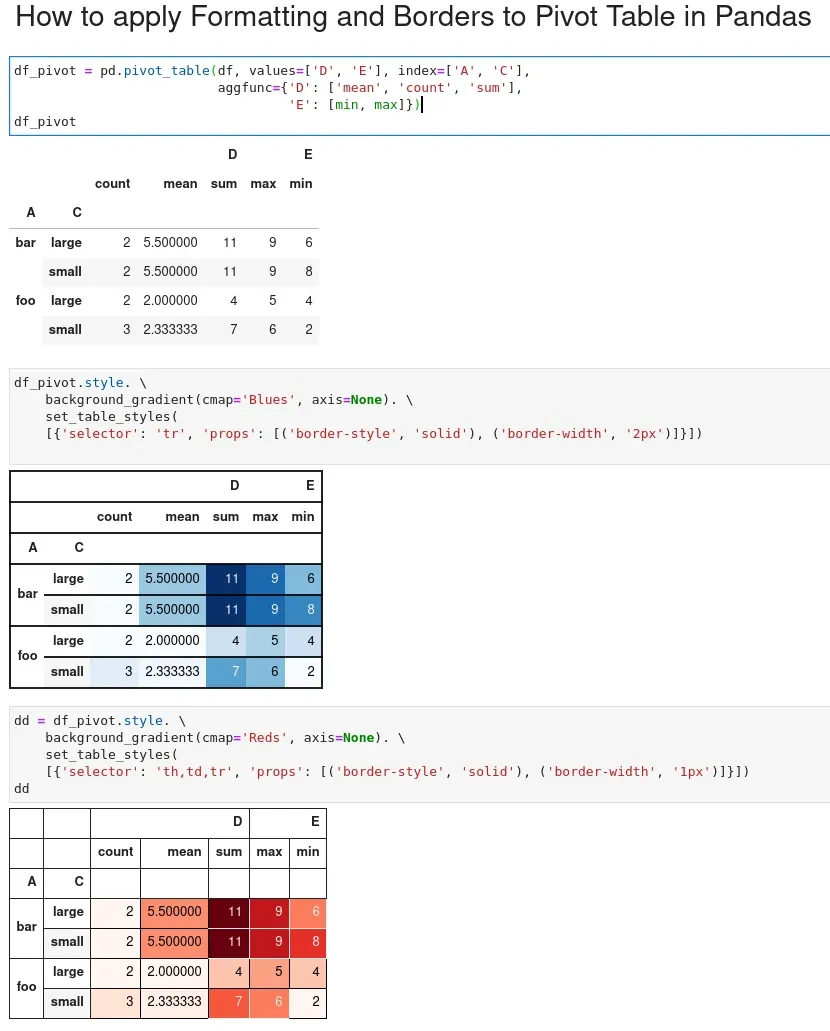To apply formatting and add borders to pivot tables in Pandas we can use `style` and `set_table_styles`.

You can find basic example on adding borders and formatting:

``````df_pivot.style. \
set_table_styles(
[{'selector': 'th,td,tr', 'props': [('border-style', 'solid'), ('border-width', '1px')]}])
``````

The result with formatting and borders of the pivot table is below:In this short post, we'll see several examples of applying formatting and adding borders to Pandas DataFrame.

## Setup

Let's say that we have the following data:

``````import pandas as pd

data = {"A": ["foo", "foo", "foo", "foo", "foo",
"bar", "bar", "bar", "bar"],
"B": ["one", "one", "one", "two", "two",
"one", "one", "two", "two"],
"C": ["small", "large", "large", "small",
"small", "large", "small", "small",
"large"],
"D": [1, 2, 2, 3, 3, 4, 5, 6, 7],
"E": [2, 4, 5, 5, 6, 6, 8, 9, 9]}

df = pd.DataFrame(data)
``````

DataFrame looks like:

A B C D E
0 foo one small 1 2
1 foo one large 2 4
2 foo one large 2 5
3 foo two small 3 5
4 foo two small 3 6
5 bar one large 4 6
6 bar one small 5 8
7 bar two small 6 9
8 bar two large 7 9

we will create pivot table by:

``````df_pivot = pd.pivot_table(df, values=['D', 'E'], index=['A', 'C'],
aggfunc={'D': ['mean', 'count', 'sum'],
'E': [min, max]})
df_pivot
``````

## Apply formatting to pivot table

To apply formatting to a pivoted DataFrame in Pandas, we can use the `style` property. In addition we can use the functions:

• `background_gradient`
• `format`
• `highlight_null`
• `set_caption`

to control the formatting of the DataFrame.

Here's an example of how to apply:

• highlight NaN values in red:
• add title to pivot DataFrame
• use brown for the column names
``````df_pivot.style.background_gradient(cmap='summer')\
.format('{:.2f}')\
.highlight_null(null_color='red')\
.set_table_styles([{'selector': 'th', 'props': [('color', 'brown')]}])\
.set_caption('Pivot with formatting')
``````

The pivot table with formatting looks like:## Add borders to pivot table

Let's say that we would like to add borders to the pivot table. We are going to use `style` and `set_table_styles`. We can use CSS selectors:

• `th` - header cell in a table
• `tr` - row in a table
• `td` - cell in a table

In this example you can find different border styles applied on each selector:

``````df_pivot.style. \
set_table_styles([{'selector': 'tr',
'props': [('border', '4px solid blue')]},
{'selector': 'th',
'props': [('border', '3px solid red')]},
{'selector': 'td',
'props': [('border', '5px solid black')]}])

``````

Running the code will give as this table:## Summary

In this post, we saw how to apply formatting, table styles and borders to pivot tables in Pandas. The correct formatting and borders will make your pivot tables easier to digest.

As you can see from the image below by default pivot formatting makes the table hard to read: